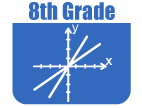IntroductionIn Grade 8, instructional time should focus on three critical areas: (1) formulating and reasoning about expressions and equations, including modeling an association in bivariate data with a linear equation, and solving linear equations and systems of linear equations; (2) grasping the concept of a function and using functions to describe quantitative relationships; (3) analyzing two- and three-dimensional space and figures using distance, angle, similarity, and congruence, and understanding and applying the Pythagorean Theorem.

(1) Students use linear equations and systems of linear equations to represent, analyze, and solve a variety of problems. Students recognize equations for proportions (y/x = m or y = mx) as special linear equations (y = mx + b), understanding that the constant of proportionality (m) is the slope, and the graphs are lines through the origin. They understand that the slope (m) of a line is a constant rate of change, so that if the input or x-coordinate changes by an amount A, the output or y-coordinate changes by the amount m·A. Students also use a linear equation to describe the association between two quantities in bivariate data (such as arm span vs. height for students in a classroom). At this grade, fitting the model, and assessing its fit to the data are done informally. Interpreting the model in the context of the data requires students to express a relationship between the two quantities in question and to interpret components of the relationship (such as slope and y-intercept) in terms of the situation.

Students strategically choose and efficiently implement procedures to solve linear equations in one variable, understanding that when they use the properties of equality and the concept of logical equivalence, they maintain the solutions of the original equation. Students solve systems of two linear equations in two variables and relate the systems to pairs of lines in the plane; these intersect, are parallel, or are the same line. Students use linear equations, systems of linear equations, linear functions, and their understanding of slope of a line to analyze situations and solve problems.

(2) Students grasp the concept of a function as a rule that assigns to each input exactly one output. They understand that functions describe situations where one quantity determines another. They can translate among representations and partial representations of functions (noting that tabular and graphical representations may be partial representations), and they describe how aspects of the function are reflected in the different representations.

(3) Students use ideas about distance and angles, how they behave under translations, rotations, reflections, and dilations, and ideas about congruence and similarity to describe and analyze two-dimensional figures and to solve problems. Students show that the sum of the angles in a triangle is the angle formed by a straight line, and that various configurations of lines give rise to similar triangles because of the angles created when a transversal cuts parallel lines. Students understand the statement of the Pythagorean Theorem and its converse, and can explain why the Pythagorean Theorem holds, for example, by decomposing a square in two different ways. They apply the Pythagorean Theorem to find distances between points on the coordinate plane, to find lengths, and to analyze polygons. Students complete their work on volume by solving problems involving cones, cylinders, and spheres.The Online Core Resource pages are a collaborative project between the Utah State Board of Education and the Utah Education Network. If you would like to recommend a high quality resource, contact Trish French (Elementary) or Lindsey Henderson (Secondary). If you find inaccuracies or broken links contact resources@uen.org.